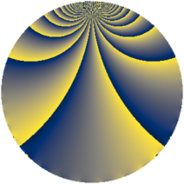# Properties

 Label 1148.2.bfLevel $1148$ Weight $2$ Character orbit 1148.bf Rep. character $\chi_{1148}(139,\cdot)$ Character field $\Q(\zeta_{10})$ Dimension $656$ Sturm bound $336$

# Learn more about

## Defining parameters

 Level: $$N$$ $$=$$ $$1148 = 2^{2} \cdot 7 \cdot 41$$ Weight: $$k$$ $$=$$ $$2$$ Character orbit: $$[\chi]$$ $$=$$ 1148.bf (of order $$10$$ and degree $$4$$) Character conductor: $$\operatorname{cond}(\chi)$$ $$=$$ $$1148$$ Character field: $$\Q(\zeta_{10})$$ Sturm bound: $$336$$

## Dimensions

The following table gives the dimensions of various subspaces of $$M_{2}(1148, [\chi])$$.

Total New Old
Modular forms 688 688 0
Cusp forms 656 656 0
Eisenstein series 32 32 0

## Trace form

 $$656q - 6q^{2} - 6q^{4} - 18q^{8} + 592q^{9} + O(q^{10})$$ $$656q - 6q^{2} - 6q^{4} - 18q^{8} + 592q^{9} - 6q^{14} - 6q^{16} - 36q^{18} - 32q^{21} + 10q^{22} + 136q^{25} - 32q^{28} - 36q^{29} - 72q^{30} + 24q^{32} - 8q^{36} - 12q^{37} - 28q^{42} - 32q^{44} + 16q^{46} + 2q^{49} - 60q^{50} - 12q^{53} - 35q^{56} - 48q^{57} + 30q^{58} - 14q^{60} + 102q^{64} - 36q^{65} + 39q^{70} - 138q^{72} - 34q^{77} - 106q^{78} + 320q^{81} - 90q^{84} - 88q^{85} - 72q^{86} - 44q^{88} + 86q^{92} - 24q^{93} + 49q^{98} + O(q^{100})$$

## Decomposition of $$S_{2}^{\mathrm{new}}(1148, [\chi])$$ into newform subspaces

The newforms in this space have not yet been added to the LMFDB.